# Formats in Perl

Formats are the writing templates used in Perl to output the reports. Perl has a mechanism which helps in generating simple reports and charts. Instead of executing, Formats are declared, so they may occur at any point in the program. Formats have their own namespace apart from the other types in Perl i.e. function named “tron” is not same as format named “tron”. However, name of the filehandle in the program is the default name for the format associated with that filehandle.

### Defining a Format

Syntax to define a Perl Format:

```format FormatName =
fieldline
value_1, value_2, value_3
fieldline
value_1, value_2, value_3
.
```

– Name of the format is denoted by the FormatName.
Fieldline is a particular way used to format the data. Fieldline can also hold text or fieldholders.
Value lines denotes/describes the values which will be entered into the fieldlines.
– Format is ended by a single period (.)
Fieldholders have the space for the data which will be entered later.

Syntax for Fieldholders:

```@<<<<<<<       left-justified (with 7 field spaces by counting '@' and '<')
@|||||||       center-justified
@###.####      numeric fieldholder
@*             multiline fieldholder
```

### Using a Format

`Write` keyword is used to call on the format declaration.

`Write FormatName;`

Format name is the name of an open file handle and the write statement sends the output to the same file handle. In order to send the data to STDOUT, format name needs to be associated with the STDOUT file handle.
Note: Use the `select()` function for making sure that STDOUT is the selected file handle.

`select (STDOUT);`

In order to associate format name with STDOUT by setting the new format name with STDOUT, use the variables like \$~ or \$Format_Name

`\$~ = "Format_Name";`

Note: For writing report in any other file handle apart from the STDOUT, use the `select()` function to select that file handle.

Example:
Input: Using STDOUT.

 `#!/usr/bin/perl ` `print` `"Content-type: text/html\n\n"``; ` `  `  `@First_Name` `= ``qw(Karthik Raman Shivam Tarun)``; ` `@Last_Name` `= ``qw(Singh Biswas Anand Mishra)``; ` `@ID` `= ``qw(101 186 009 359)``; ` `@Phone_Number` `= ``qw(963x 885x 734x 921x)``; ` `  `  `format` `DATA =  ` `@<<<<<<<<<<<<<<<@<<<<<<<<<<<<<<<@<<<<<<<<<<<<<<<@<<<<<<<<<<<<<<< ` `\$First_Name``[``\$k``] ``\$Last_Name``[``\$k``]  ``\$ID``[``\$k``]         ``\$Phone_Number``[``\$k``] ` `  `  `. ` `  `  `select``(STDOUT); ` `\$~ = DATA; ` `\$k` `= 0; ` `while` `(``\$k` `<= ``\$#First_Name``) ` `{ ` `    ``write``; ` `    ``\$k``++; ` `} `

Output :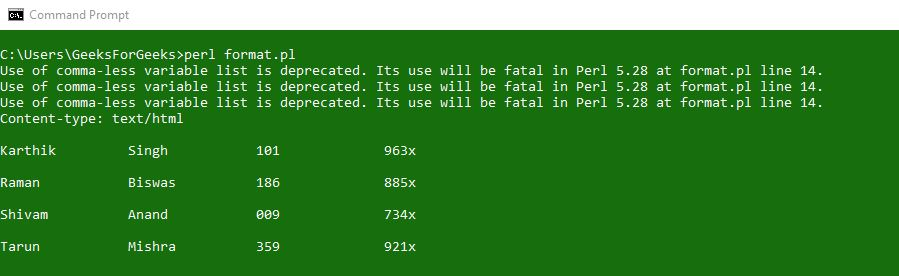Input: Using other file handle(Printing the output into a text file.)

 `#!/usr/bin/perl ` ` `  `print` `"Content-type: text/html\n\n"``; ` ` `  ` `  `@First_Name` `= ``qw(Karthik Raman Shivam Tarun)``; ` `@Last_Name` `= ``qw(Singh Biswas Anand Mishra)``; ` `@ID` `= ``qw(101 186 009 359)``; ` `@Phone_Number` `= ``qw(963x 885x 734x 921x)``; ` ` `  ` `  `format` `DATA =  ` `@<<<<<<<<<<<<<<<@<<<<<<<<<<<<<<<@<<<<<<<<<<<<<<<@<<<<<<<<<<<<<< ` `\$First_Name``[``\$k``] ``\$Last_Name``[``\$k``]  ``\$ID``[``\$k``]         ``\$Phone_Number``[``\$k``] ` ` `  `. ` ` `  `open``(FILE, ``">file.txt"``); ` `select` `FILE; ` `\$~ = DATA; ` ` `  `\$k` `= 0; ` `while` `(``\$k` `<= ``\$#First_Name``) ` `{ ` `    ``write``; ` `    ``\$k``++; ` `} ` ` `  `close` `FILE; `

Output :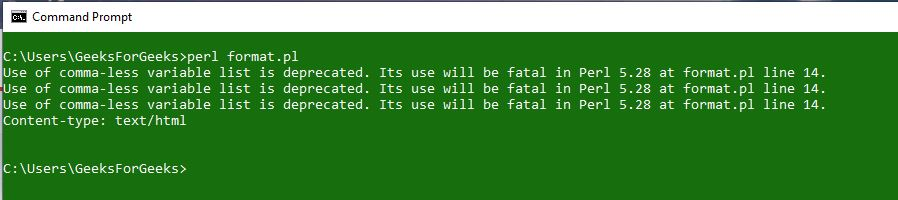File where data is printed: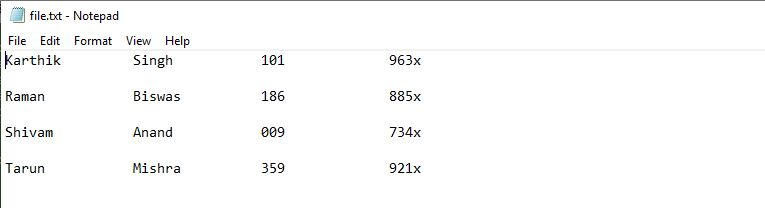Header is something that appears at the top of each page. Instead of defining a template, just define a header and assign it to \$^ or \$FORMAT_NAME_TOP.
Footer has a fixed size. It can be achieved by checking for variable \$-. You can even print the footer by yourself if necessary using the syntax given below,

```format FORMAT_NAME_BOTTOM
End of Page \$%
```

Example:
Input: Using STDOUT

 `#!/usr/bin/perl ` `print` `"Content-type: text/html\n\n"``; ` `  `  `format` `DATA_TOP =  ` `@|||||||||||||||||||||||||||||||||||||||||||||||||||| ` `                  ``"Staff Members"` `               ``---------------------- ` `First           Last            ID              Phone ` `------------------------------------------------------ ` `. ` `  `  `@First_Name` `= ``qw(Karthik Raman Shivam Tarun)``; ` `@Last_Name` `= ``qw(Singh Biswas Anand Mishra)``; ` `@ID` `= ``qw(101 186 009 359)``; ` `@Phone_Number` `= ``qw(963x 885x 734x 921x)``; ` `  `  `format` `DATA =  ` `@<<<<<<<<<<<<<<<@<<<<<<<<<<<<<<<@<<<<<<<<<<<<<<<@<<<<<<<<<<<<<<< ` `\$First_Name``[``\$k``] ``\$Last_Name``[``\$k``]  ``\$ID``[``\$k``]         ``\$Phone_Number``[``\$k``] ` `  `  `. ` `  `  `select` `(STDOUT); ` `\$~ = DATA; ` `\$^ = DATA_TOP; ` `\$k` `= 0; ` `while` `(``\$k` `<= ``\$#First_Name``) ` `{ ` `    ``write``; ` `    ``\$k``++; ` `} `

Output: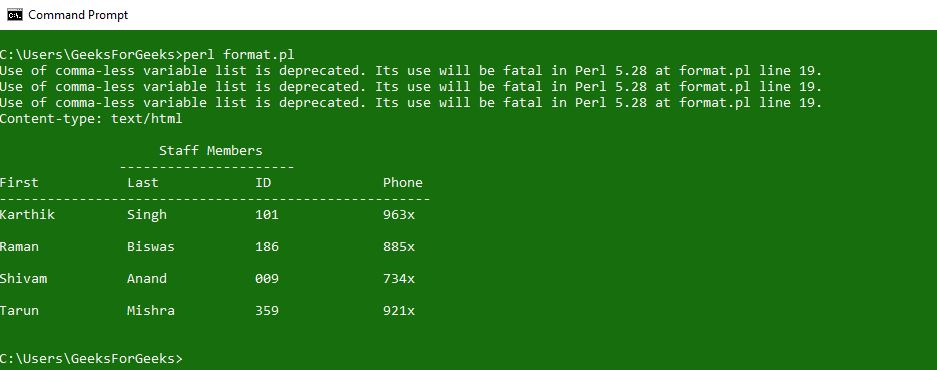Input: Getting output into a text file.

 `#!/usr/bin/perl ` `print` `"Content-type: text/html\n\n"``; ` ` `  `format` `DATA_TOP =  ` `@|||||||||||||||||||||||||||||||||||||||||||||||||||| ` `                  ``"Staff Members"` `               ``---------------------- ` `First           Last            ID              Phone ` `------------------------------------------------------ ` `. ` ` `  `@First_Name` `= ``qw(Karthik Raman Shivam Tarun)``; ` `@Last_Name` `= ``qw(Singh Biswas Anand Mishra)``; ` `@ID` `= ``qw(101 186 009 359)``; ` `@Phone_Number` `= ``qw(963x 885x 734x 921x)``; ` ` `  ` `  `format` `DATA =  ` `@<<<<<<<<<<<<<<<@<<<<<<<<<<<<<<<@<<<<<<<<<<<<<<<@<<<<<<<<<<<<<< ` `\$First_Name``[``\$k``] ``\$Last_Name``[``\$k``]  ``\$ID``[``\$k``]         ``\$Phone_Number``[``\$k``] ` ` `  `. ` ` `  `open` `(FILE, ``">file.txt"``); ` `select` `FILE; ` `\$~ = DATA; ` `\$^ = DATA_TOP; ` ` `  `\$k` `= 0; ` `while` `(``\$k` `<= ``\$#First_Name``) ` `{ ` `    ``write``; ` `    ``\$k``++; ` `} ` `close` `FILE; `

Output: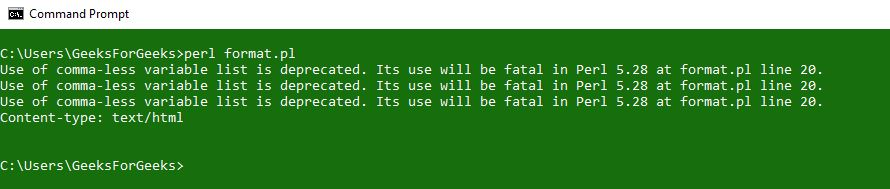File where data is printed: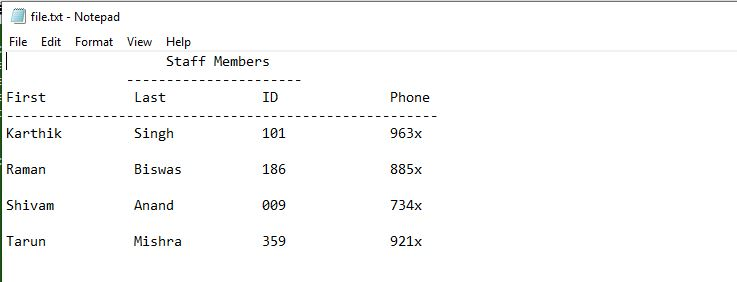### Pagination

Pagination comes into the picture when you have a long report which will not fit in a single page. Use of variables like \$% or \$FORMAT_PAGE_NUMBER along with the header in the format helps in defining the page number to more than one page. Default number of lines in a page is 60 but it can be set manually too by using the variables \$= or \$FORMAT_LINES_PER_PAGE.

Example:

 `#!/usr/bin/perl ` `print` `"Content-type: text/html\n\n"``; ` ` `  `format` `DATA_TOP =  ` `@>>>>>>>>>>>>>>>>>>>>>>>>>>>>>>>>>>>>>>>>>>>>>>>>>>>> ` `"[Page \$%]"` `@|||||||||||||||||||||||||||||||||||||||||||||||||||| ` `                  ``"Staff Members"` `               ``---------------------- ` `First           Last            ID              Phone ` `------------------------------------------------------ ` `. ` ` `  `@First_Name` `= ``qw(Karthik Raman Shivam Tarun)``; ` `@Last_Name` `= ``qw(Singh Biswas Anand Mishra)``; ` `@ID` `= ``qw(101 186 009 359)``; ` `@Phone_Number` `= ``qw(963x 885x 734x 921x)``; ` ` `  ` `  `format` `DATA =  ` `@<<<<<<<<<<<<<<<@<<<<<<<<<<<<<<<@<<<<<<<<<<<<<<<@<<<<<<<<<<<<<<< ` `\$First_Name``[``\$k``] ``\$Last_Name``[``\$k``]  ``\$ID``[``\$k``]         ``\$Phone_Number``[``\$k``] ` ` `  `. ` ` `  `select` `(STDOUT); ` `\$~ = DATA; ` `\$^ = DATA_TOP; ` `\$= = 1; ` ` `  `\$k` `= 0; ` `while` `(``\$k` `<= ``\$#First_Name``) ` `{ ` `    ``write``; ` `    ``\$k``++; ` `} `

Output: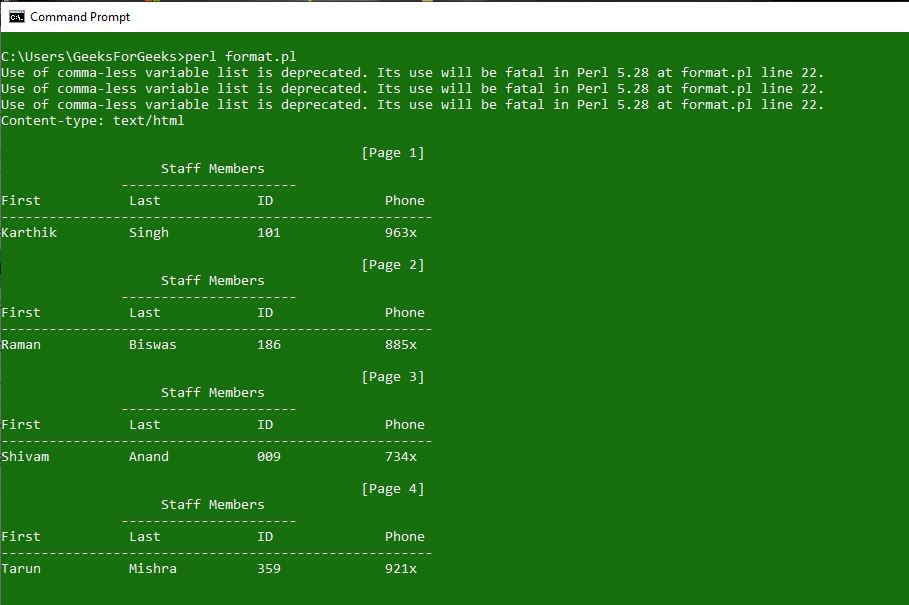My Personal Notes arrow_drop_upCheck out this Author's contributed articles.

If you like GeeksforGeeks and would like to contribute, you can also write an article using contribute.geeksforgeeks.org or mail your article to contribute@geeksforgeeks.org. See your article appearing on the GeeksforGeeks main page and help other Geeks.

Please Improve this article if you find anything incorrect by clicking on the "Improve Article" button below.

Article Tags :
Practice Tags :

Be the First to upvote.

Please write to us at contribute@geeksforgeeks.org to report any issue with the above content.